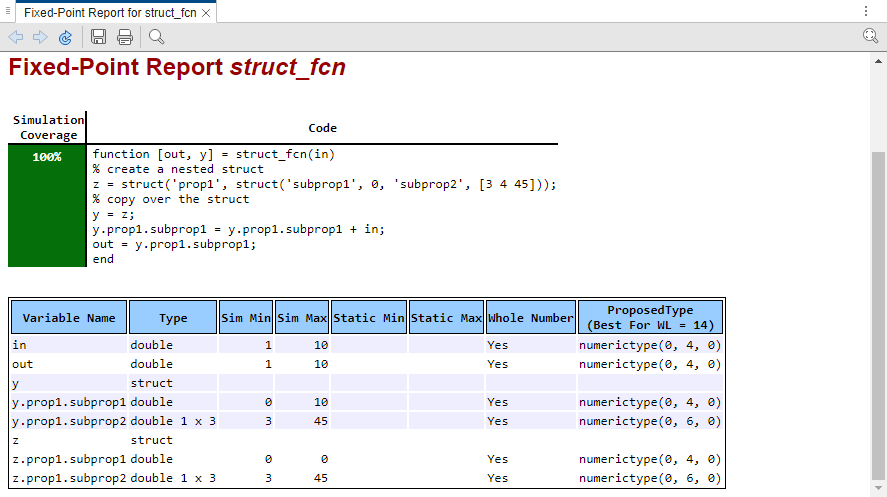## Convert Code Containing Structures to Fixed Point

This example shows how to convert a MATLAB® algorithm containing structures to fixed point using the Fixed-Point Converter app.

1. In a local writable folder, create the functions `struct_fcn.m`

```function [out, y] = struct_fcn(in) % create a nested struct z = struct('prop1', struct('subprop1', 0, 'subprop2', [3 4 45])); % copy over the struct y = z; y.prop1.subprop1 = y.prop1.subprop1 + in; out = y.prop1.subprop1; end```

2. In the same folder, create a test file, `struct_fcn_tb.m`, that calls the function.

```for ii = 1:10 struct_fcn(ii); end```

3. From the apps gallery, open the Fixed-Point Converter app.

4. On the Select Source Files page, browse to the `struct_fcn.m` file, and click .

5. Click . On the Define Input Types page, enter the test file that exercises the `struct_fcn` function. Browse to select the `struct_fcn_tb.m` file. Click .

6. Click . The app generates an instrumented MEX function for your entry-point MATLAB function. On the Convert to Fixed-Point page, click Simulate to simulate the function, gather range information, and propose data types.

When the names, number, and types of fields of two or more structures match, the Fixed-Point Converter app proposes a unified type. In this example, the range of `z.prop1.subprop1` is `[0,0]`, while the range of `y.prop1.subprop1` is `[0,10]`. The app proposes a data type of `numerictype(0,4,0)` for both `z.prop1.subprop1` and `y.prop1.subprop1` based on the union of the ranges of the two fields.7. Click Convert.

The Fixed-Point Converter converts the function containing the structures to fixed point and generates the `struct_fcn_fixpt.m` file.##### SupportGet trial now